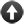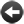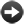§15.7. Trigonometry

We have twelve functions left to cover, though they are all closely related.

(real number) degrees ... real number

Inform measures angles in radians, a convention in which the angle for a half circle is pi, and a right angle is pi divided by 2. This is better from a mathematical point of view, but in practice most people think about angles using degree , where 180 degrees is a half-circle and a right angle is 90 degrees. This phrase helps with that by converting from degrees to radians: in other words, it multiplies by 0.0174532925, since that's roughly 1/180th of pi. Examples:

sine of 90 degrees = 0.0
cosine of 60 degrees = 0.5

sine of (real number) ... real number

The length of the upright of a right-angled triangle with this angle and a hypotenuse of length 1, where angle is measured in radians. Examples:

sine of 0 = 0
sine of 45 degrees = 0.70711
sine of (pi divided by 4) = 0.70711
sine of (pi divided by 2) = 1.0
sine of pi = 0

cosine of (real number) ... real number

The length of the base of a right-angled triangle with this angle and a hypotenuse of length 1, where angle is measured in radians. Examples:

cosine of 0 = 1.0
cosine of 45 degrees = 0.70711
cosine of (pi divided by 4) = 0.70711
cosine of (pi divided by 2) = 0.0
cosine of pi = -1.0

tangent of (real number) ... real number

The ratio of the upright length to the base length in a right-angled triangle with this angle and a hypotenuse of length 1, where angle is measured in radians. Examples:

tangent of 0 = 0.0
tangent of 45 degrees = 1.0
tangent of (pi divided by 4) = 1.0
tangent of (pi divided by 2) = plus infinity

arcsine of (real number) ... real number

The inverse of the sine function.

arccosine of (real number) ... real number

The inverse of the cosine function.

arctangent of (real number) ... real number

The inverse of the tangent function.

hyperbolic sine of (real number) ... real number

The hyperbolic sine function, often written "sinh" but pronounced "shine".

hyperbolic cosine of (real number) ... real number

The hyperbolic cosine function, often written "cosh".

hyperbolic tangent of (real number) ... real number

The hyperbolic tangent function, often written "tanh".

hyperbolic arcsine of (real number) ... real number

The inverse of the hyperbolic sine function.

hyperbolic arccosine of (real number) ... real number

The inverse of the hyperbolic cosine function.

hyperbolic arctangent of (real number) ... real number

The inverse of the hyperbolic tangent function.Start of Chapter 15: Numbers and EquationsBack to §15.6. Powers and logarithmsOnward to §15.8. Units